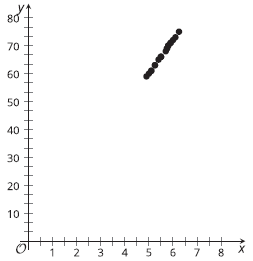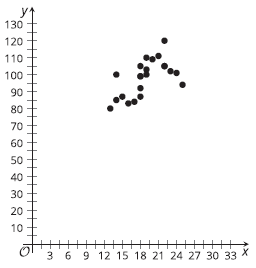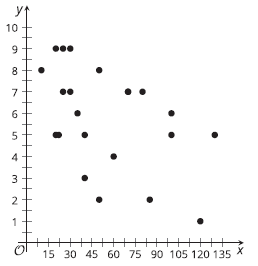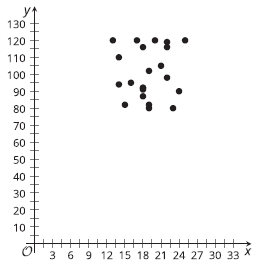# A.3.8.1 Putting the Numbers in Context

Topic:
Numbers
For each graph below, match the variables to the scatter plot you think they best fit. (think about how strongly the variables might be related and then how close to the line the data values would be).Select all that apply
• A
• B
• C
• DSelect all that apply
• A
• B
• C
• DSelect all that apply
• A
• B
• C
• DSelect all that apply
• A
• B
• C
• D